#Repeated EigenvaluesConsider the linear homogeneous system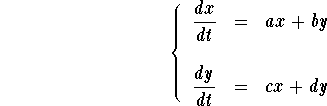In order to find the eigenvalues consider the Characteristic polynomial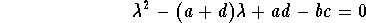In this section, we consider the case when the above quadratic equation has double real root (that is if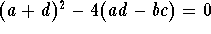) the double root (eigenvalue) is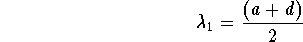In this case, we know that the differential system has the straight-line solution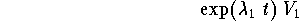where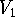is an eigenvector associated to the eigenvalue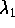. We also know that the general solution (which describes all the solutions) of the system will be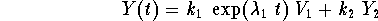where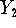is another solution of the system which is linearly independent from the straight-line solution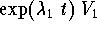. Therefore, the problem in this case is to find.

Search for a second solution.

Let us use the vector notation. The system will be written as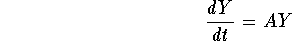where A is the matrix coefficient of the system. Write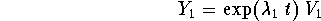The idea behind finding a second solution, linearly independent from, is to look for it as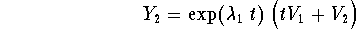where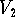is some vector yet to be found. Since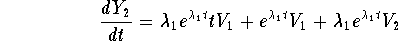and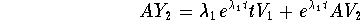(where we used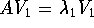), then (becauseis a solution of the system) we must have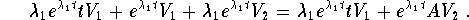Simplifying, we obtain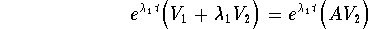or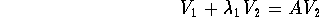This equation will help us find the vector. Note that the vectorwill automatically be linearly independent from(why?). This will help establish the linear independence offrom.

Example. Find two linearly independent solutions to the linear system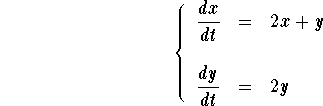Answer. The matrix coefficient of the system is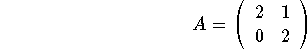In order to find the eigenvalues consider the Characteristic polynomial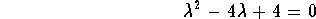Since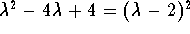, we have a repeated eigenvalue equal to 2. Let us find the associated eigenvector. Set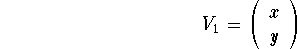Then we must have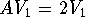which translates into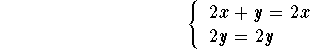This reduces to y=0. Hence we may take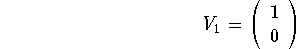Next we look for the second vector. The equation giving this vector is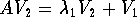which translates into the algebraic system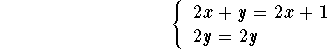where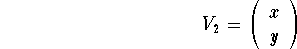Clearly we have y=1 and x may be chosen to be any number. So we take x=0 for example to get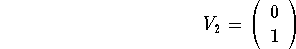Therefore the two independent solutions are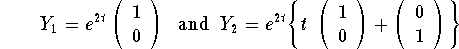The general solution will then be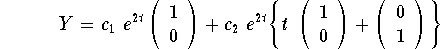Qualitative Analysis of Systems with Repeated Eigenvalues

Recall that the general solution in this case has the form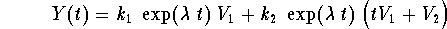where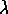is the double eigenvalue andis the associated eigenvector. Let us focus on the behavior of the solutions when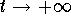(meaning the future). We have two casesIf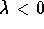, then clearly we have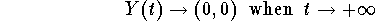In this case, the equilibrium point (0,0) is a sink. On the other hand, when t is large, we have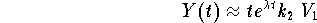So the solutions tend to the equilibrium point tangent to the straight-line solution. Note that is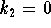, then the solution is the straight-line solution which still tends to the equilibrium point.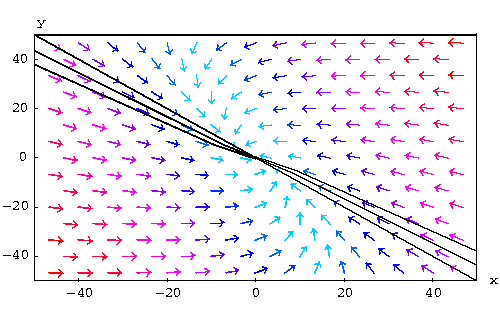If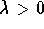, then Y(t) tends to infinity as, except of course the constant solution. Note again that if, then we are moving along the straight-line solution.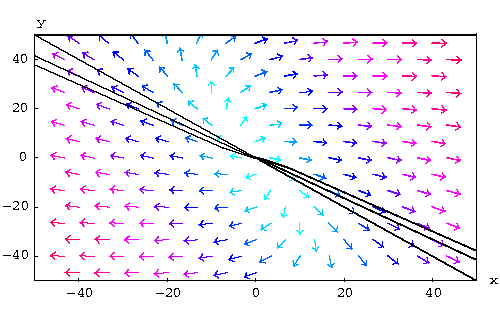Another example of the repeated eigenvalue's case is given by harmonic oscillators.

### If you would like more practice, click on Example.[Differential Equations] [First Order D.E.]
[Geometry] [Algebra] [Trigonometry ]
[Calculus] [Complex Variables] [Matrix Algebra]S.O.S MATHematics home page

Do you need more help? Please post your question on our S.O.S. Mathematics CyberBoard.Author: Mohamed Amine Khamsi